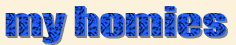MONTRÉAL HOW SPICY IS IT OUT THERE?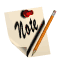Theorem A (Alon). Let $k$ be a field and let $f\in k[x_1,x_2\ldots,x_n]$ be a polynomial of degree $d$. If the coefficient of $x_1^{d_1}x_2^{d_2}\cdots x_n^{d_n}$ is nonzero for $d_1 + d_2 + \cdots + d_n = d$ and $S_1, S_2, \ldots, S_n$ are subsets of $k$ such that $|S_i|>d_i$ for all $1\leq i\leq n$, then there exists $x_1\in S_1, x_2\in S_2, \ldots, x_n\in S_n$ such that $f(x_1, x_2,\ldots,x_n) \neq 0$.

For an ideal $I$ of a ring $R$, the set $\sqrt I = \{r\in R : r^m\in I\ \hbox{for some integer}\ m\}$ is called the radical of $I$. For a field $k$ and an arbitrary subset $I\subseteq k[x_1,x_2,\ldots,x_n]$, the corresponding algebraic subset is the set of all $(a_1, a_2, \ldots,a_n)\in k^n$ such that $f(a_1,a_2,\ldots,a_n)=0$ for all $f\in k[x_1,x_2,\ldots,x_n]$. For any subset $X$ of $k^n$, we define $I(X)$ to be the set of all $f\in k[x_1,x_2,\ldots,x_n]$ such that $f(a_1,a_2,\ldots,x_n)=0$ for all $(a_1,a_2,\ldots,a_n) \in k^n$.

Theorem H (Hilbert). Let $k$ be an algebraically closed field and let $I$ be an ideal of $k[x_1, x_2,\ldots,x_n]$. Then $I(Z(I)) = \sqrt I$ and the correspondences $I\mapsto Z(I)$ and $X\mapsto I(X)$ induce a bijection between the algebraic subsets of $k^n$ and the radical ideals of $k[x_1,x_2,\ldots,x_n]$.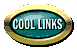##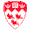McGill

𓅓 MinervamyCoursesVSB 𓁼 Lecture recordingsMcGill E-Mailr/mcgill

##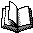Research

arXivGoogle ScholarResearchGate 𐂷 Mathematics genealogyCollaboration distance calculatorMathSciNet

##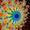Mathematics

DesmosOEISOverleafRichard E. Borcherds lecturesTimothy Gowers lecturesGraph Theory and Additive Combinatorics lecturesTerry Tao's blogGowers's weblogQuanta

##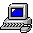Computer Science

EWD Archive 𝛌 SICP lecturesKnuth lectures 𓆛 Beluga repository

##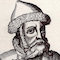Typesetting

CM fontsCM font tablesTeX cheat sheetPostScript commands

##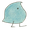Linguistics

IPA chartWiktionarySLUM Rocket.Chat

##Reviews

BooksGames I've finishedRecipesOld articles (2011-2017)

##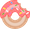Other